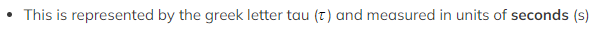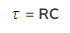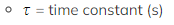# CIE A Level Physics复习笔记19.2.2 Capacitor Discharge Equations

### The Time Constant

• The time constant of a capacitor discharging through a resistor is a measure of how long it takes for the capacitor to discharge
• The definition of the time constant is:

The time taken for the charge of a capacitor to decrease to 0.37 of its original value

•The time constant gives an easy way to compare the rate of change of similar quantities eg. charge, current and p.d.
• The time constant is defined by the equation:
•Where:
•R = resistance of the resistor (Ω)
• C = capacitance of the capacitor (F)The graph of voltage-time for a discharging capacitor showing the positions of the first three time constants

#### Worked Example

A capacitor of 7 nF is discharged through a resistor of resistance R. The time constant of the discharge is 5.6 × 10-3 s.Calculate the value of R.

Step 1: Write out the known quantities

Capacitance, C = 7 nF = 7 × 10-9 F

Time constant, τ = 5.6 × 10-3 s

Step 2: Write down the time constant equation

τ = RC

Step 3: Rearrange for resistance RStep 4: Substitute in values and calculate### Using the Capacitor Discharge Equation

• The time constant is used in the exponential decay equations for the current, charge or potential difference (p.d) for a capacitor discharging through a resistor
• These can be used to determine the amount of current, charge or p.d left after a certain amount of time when a capacitor is discharging
• The exponential decay of current on a discharging capacitor is defined by the equation:• Where:
• I = current (A)
• I0 = initial current before discharge (A)
• e = the exponential function
• t = time (s)
• RC = resistance (Ω) × capacitance (F) = the time constant τ (s)
• This equation shows that the faster the time constant τ, the quicker the exponential decay of the current when discharging
• Also, how big the initial current is affects the rate of discharge
• If I0 is large, the capacitor will take longer to discharge
• Note: during capacitor discharge, I0 is always larger than I, this is because the current I will always be decreasing
• The current at any time is directly proportional to the p.d across the capacitor and the charge across the parallel plates
• Therefore, this equation also describes the change in p.d and charge on the capacitor:• Where:
• Q = charge on the capacitor plates (C)
• Q0 = initial charge on the capacitor plates (C)• Where:
• V = p.d across the capacitor (C)
• V0 = initial p.d across the capacitor (C)

#### The Exponential Function e

• The symbol e represents the exponential constant, a number which is approximately equal to e = 2.718...
• On a calculator it is shown by the button ex
• The inverse function of ex is ln(y), known as the natural logarithmic function
• This is because, if ex = y, then x = ln (y)
• The 0.37 in the definition of the time constant arises as a result of the exponential constant, the true definition is:#### Worked Example

The initial current through a circuit with a capacitor of 620 μF is 0.6 A. The capacitor is connected across the terminals of a 450 Ω resistor.Calculate the time taken for the current to fall to 0.4 A.

Step 1: Write out the known quantities

Initial current before discharge, I0 = 0.6 A

Current, I = 0.4 A

Resistance, R = 450 Ω

Capacitance, C = 620 μF = 620 × 10-6 F

Step 2: Write down the equation for the exponential decay of currentStep 3: Calculate the time constant

τ = RC

τ = 450 × (620 × 10-6) = 0.279 s

Step 4: Substitute into the current equationStep 5: Rearrange for the time tThe exponential can be removed by taking the natural log of both sides:#### Exam Tip

Make sure you’re confident in rearranging equations with natural logs (ln) and the exponential function (e). To refresh your knowledge of this, have a look at the AS Maths revision notes on Exponentials & Logarithms